# MCQs on Electric Circuits

##### Page 23 of 63. Go to page 1 2 3 4 5 6 7 8 9 10 11 12 13 14 15 16 17 18 19 20 21 22 23 24 25 26 27 28 29 30 31 32 33 34 35 36 37 38 39 40 41 42 43 44 45 46 47 48 49 50 51 52 53 54 55 56 57 58 59 60 61 62 63
01․ Which variety of copper has the best mechanical strength ?
Annealed copper.
Hard drawn copper.
Cast copper.
Soft copper.

Hard drawn copper is not annealed after the drawing process. Annealing makes the copper more flexible. Hard drawn has at least 150 % more tensile strength than annealed.

02․ What will be the resistance if 10 resistors of 10 ohm each are connected in series?
100 Ω.
1 Ω.
0.1 Ω.
10 Ω.

Equivalent resistance of resistors connected in series = sum of the individual resistances. Here it is 10 + 10 + 10 + 10 + 10 + 10 + 10 + 10 + 10 + 10 = 100 Ω.

03․ If the three colour bands of a resistor are grey, violet and gold, what is the value of the resistor?
6.7 ohms.
8.7 ohms.
7.7 ohms.
9.7 ohms.

The values of these colours are 8, 7 and multiplier of 0.1. Therefore, the value of resistance will be (87 × 0.1) = 8.7 ohms.

04․ Which of the following may be the value of resistivity of copper at absolute zero in ohm-metre?
0.020.
3.12.
6.24.
1.56.

The resistivity of copper does not vanish at absolute zero. Instead, its level at absolute zero is known as the residual resistance. Copper has a residual resistance of 0.020 Ω - m.

05․ A circuit contains two unequal resistor in parallel
voltage drops across both are same.
currents in both are same.
heat losses in both are same.
voltage drops are according to their resistive value.

Whatever may be the value of resistance the voltage drops, across all the resistors connected in parallel, are always same.

06․ Three resistances have the following ratings 150 Ω at 5 %, 100 Ω at 5 % and 200 Ω at 5 % respectively. The percentage error when all the three are connected in series will be
+ 6 %.
+ 5 %.
+ 7 %.
+ 8 %.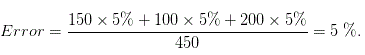07․ If the length of a wire of resistance R is uniformly stretched to n times its original value, what will be its new resistance
n2R.
R / n2.
n2 / R.
nR .

Length of the conductor becomes n times and at the same time cross section of the conductor becomes 1 / nth times. Hence resistance of the conductor becomes n2 times of its original value as length of the conductor is directly proportional and cross section is inversely proportional to the resistance.

08․ The resistivity of semi-conductors at room temperatures is
0.01 to 50 ohm - cm.
1000 to 1500 ohm - cm.
109 to 1012 ohm - cm.
1.6 × 106 to 100 × 106 ohm - cm.

The resistivity of semi-conductors at room temperatures is 0.01 to 50 ohm - cm.

09․ The current in the resistor R shown in figure will be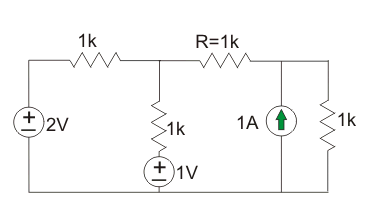0.2 A.
0.4 A.
0.6 A.
0.8 A.

Applying superposition principle by 1st considering 2 V and 1 V source and then considering 1 Amp source.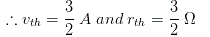Current through r, ith = 3 / 5 A. Now considering 1 amp source, current through R = 2 / 5 A. Net current through R is equal to ( 1 / 5 ) = 0.2 A.

10․ In given figure, the value of resistance R in Ω is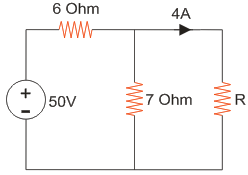3.5 Ω
2.5 Ω
1 Ω
4.5 Ω

The given circuit can be simplified by replacing voltage source by equivalent current source as below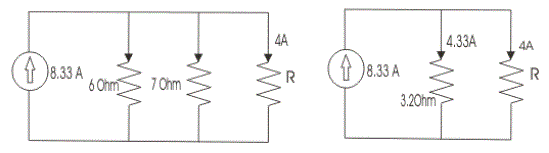The current through 3.2 Ω resistor is 4.33 A hence voltage across it is 3.2 X 4.33 = 13.856 V and this is the voltage across unknown resistor R. As current through R is 4 A and then R = 13.856 / 4 = 3.47 Ω.

<<<2122232425>>>